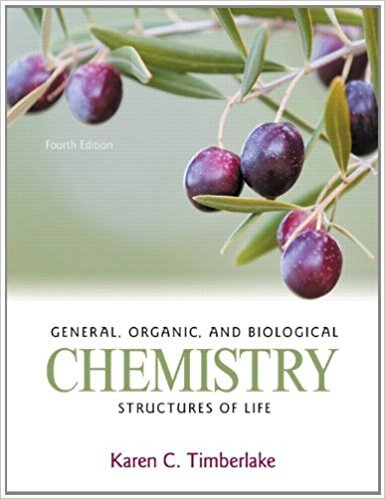×
×

# Pentane gas, C5H12, reacts with oxygen to produce carbonISBN: 9780321750891 35

## Solution for problem 117CQ Chapter 6

General, Organic, and Biological Chemistry: Structures of Life | 4th Edition

• Textbook Solutions
• 2901 Step-by-step solutions solved by professors and subject experts
• Get 24/7 help from StudySoup virtual teaching assistantsGeneral, Organic, and Biological Chemistry: Structures of Life | 4th Edition

4 5 1 357 Reviews
12
3
Problem 117CQ

Pentane gas, C5H12, reacts with oxygen to produce carbon dioxide and water. (6.6, 6.7, 6.8) a. How many grams of pentane must react to produce 4.0 moles of water?b. How many grams of CO2 are produced from 32.0 g of oxygen and excess pentane?c. How many grams of CO2 are formed if 44.5 g of C5H12 is reacted with 108 g of O2?

Step-by-Step Solution:

Solution 117CQStep 1 of 4:Given reaction: a. Here, we are asked to find the grams of pentane that must react to produce 4.0 moles of water.Molar mass of C5H12 = 72.15 g/mol. This means, 1 mol C5H12 = 72.15 g. So, the mole-mass factor is: and To produce 4.0 moles of water, 4/6 moles of pentane must be reacted. Hence, the mass of pentane that reacts is: = mol C5H12 = 48.1 g C5H12Hence, the grams of pentane that must react to produce 4.0 moles of water is 48 g ________________Step 2 of 4:b. Here, we are asked to find the grams of CO2 produced from 32.0 g of oxygen and excess pentane.Molar mass of O2 = 31.998 g/mol. This means, 1 mol O2 = 31.998 g. So, the mole-mass factor is: and In the equation, we see 5 mol CO2 = 8 mol O2. Hence, the mole-mole factor is: and Molar mass of CO2 = 44.01 g/mol. This means, 1 mol CO2 = 44.01 g. So, the mole-mass factor is: and = 32.0 g of O2 = 27.50 g CO2Hence, the grams of CO2 produced from 32.0 g of oxygen and excess pentane is 27.50 g.________________

Step 3 of 4

Step 4 of 4

##### ISBN: 9780321750891

Unlock Textbook Solution